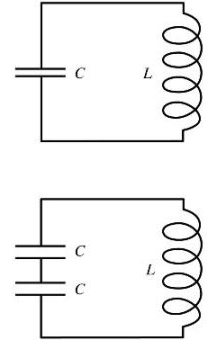# Problem: If the top circuit has an oscillation frequency of 1000 Hz, the frequency of the bottom circuit is?

###### FREE Expert Solution

Frequency of LC circuit:

$\overline{){\mathbit{f}}{\mathbf{=}}\frac{\mathbf{1}}{\mathbf{2}\mathbf{\pi }\sqrt{\mathbf{L}\mathbf{C}}}}$

For the first circuit, the frequency is expressed as:

$\begin{array}{rcl}\mathbf{f}& \mathbf{=}& \frac{\mathbf{1}}{\mathbf{2}\mathbf{\pi }}\frac{\mathbf{1}}{\sqrt{\mathbf{L}\mathbf{C}}}\\ \frac{\mathbf{1}}{\sqrt{\mathbf{L}\mathbf{C}}}& \mathbf{=}& \mathbf{2}\mathbf{\pi }\mathbf{f}\\ & \mathbf{=}& \mathbf{2}\mathbf{\pi }\mathbf{\left(}\mathbf{1000}\mathbf{\right)}\end{array}$

For the second circuit:

Equivalent capacitance for the second circuit:

$\begin{array}{rcl}\frac{\mathbf{1}}{{\mathbf{C}}_{\mathbf{e}\mathbf{q}}}& \mathbf{=}& \frac{\mathbf{1}}{\mathbf{C}}\mathbf{+}\frac{\mathbf{1}}{\mathbf{C}}\\ \frac{\mathbf{1}}{{\mathbf{C}}_{\mathbf{e}\mathbf{q}}}& \mathbf{=}& \frac{\mathbf{2}}{\mathbf{C}}\end{array}$

100% (377 ratings)###### Problem Details

If the top circuit has an oscillation frequency of 1000 Hz, the frequency of the bottom circuit is?